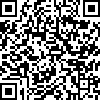TOP

2020-05-28 14:16:00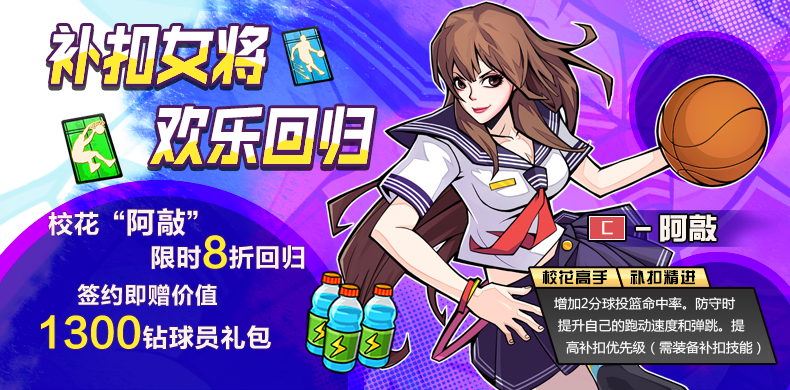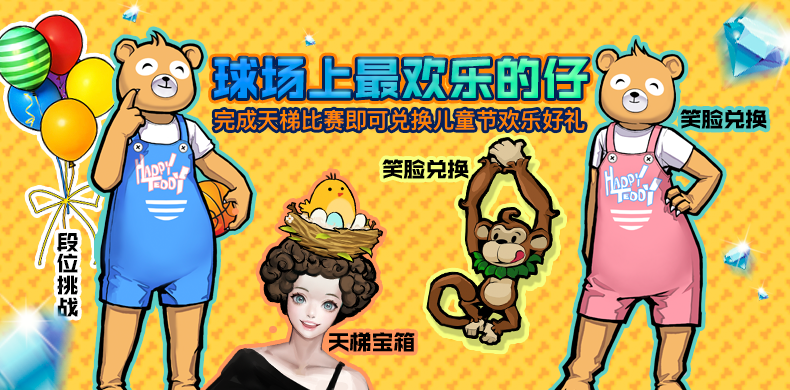=帕丁熊（男）*1 限1次

=帕丁熊（女）*1 限1次

=调皮猴项链*1 限1次

=小黄鸭*1 限1次

=儿童节宝箱*1 限10次

=笑脸*5；矿泉水大*5

=笑脸*5；特训卡资深*5

=笑脸*5；特训卡大师*2

=笑脸*5；特训卡王者*1

=钻石*58

=钻石*88

=钻石*188

=钻石*288

=魔法气球背饰*1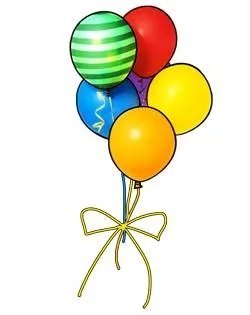*打开儿童节宝箱有几率获得“黄小鸡”头饰。

=训练球*3

=资深技能卡*3

=矿泉水*3=训练球*5

=资深技能卡*5

=矿泉水*5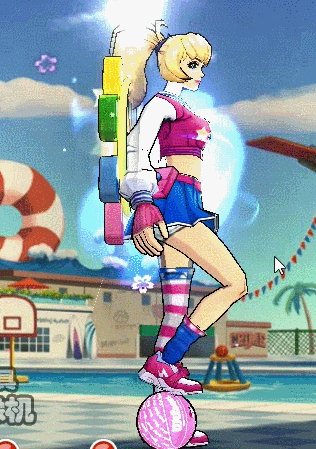*以上活动请以游戏内实际上线为准。